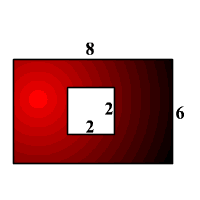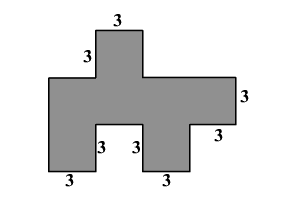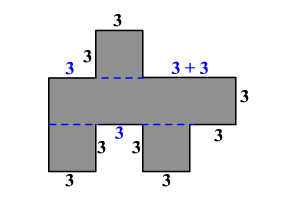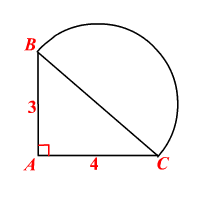# Area Problem Solving

Sometimes you can use the simple area formulas for rectangles , triangles , and circles in combination to find the areas of more complicated shapes.

Example 1:

What is the area of the shaded region?The area of the shaded region is the difference between the areas of the rectangle and the square.

The dimensions of the rectangle are $6$ by $8$ and so the area is $6$ times $8$ , that is $48$ square units.

Each side of the square measures $2$ units and so the area is $2$ times $2$ , that is $4$ square units.

Therefore, the area of the shaded region is $48-4=44$ square units.

Example 2:

Find the total area of the shaded region. (In the figure, all angles are right angles.)The given figure can be divided into $3$ squares and a rectangle as shown.The total area is the sum of the areas of the rectangle and the squares.

The dimensions of the rectangle are $3$ by $12$ and so the area is $3$ times $12$ , that is $36$ square units.

All the three squares have each side $3$ units long and so the area of each of them is $3$ times $3$ , that is $9$ square units.

Therefore, the total area is $36+\left(3×9\right)=63$ square units.

Example 3:

If $\Delta ABC$ is a right triangle and $\stackrel{⌢}{BC}$ is a semicircle, find the total area of the figure.The total area is the sum of the areas of the triangle and the semicircle.

We have the length of a side and that of the altitude to the side of the triangle. So, the area of the triangle is

Since $\stackrel{⌢}{BC}$ is a semicircle, $\stackrel{¯}{BC}$ is a diameter. But $\stackrel{¯}{BC}$ is also the hypotenuse of the right triangle $ABC$ .

Use the Pythagorean Theorem to find the length $BC$ .

$\begin{array}{l}BC=\sqrt{{3}^{2}+{4}^{2}}\\ \text{\hspace{0.17em}}\text{\hspace{0.17em}}\text{\hspace{0.17em}}\text{\hspace{0.17em}}\text{\hspace{0.17em}}\text{\hspace{0.17em}}\text{\hspace{0.17em}}\text{\hspace{0.17em}}\text{\hspace{0.17em}}=\sqrt{9+16}\\ \text{\hspace{0.17em}}\text{\hspace{0.17em}}\text{\hspace{0.17em}}\text{\hspace{0.17em}}\text{\hspace{0.17em}}\text{\hspace{0.17em}}\text{\hspace{0.17em}}\text{\hspace{0.17em}}\text{\hspace{0.17em}}=\sqrt{25}\\ \text{\hspace{0.17em}}\text{\hspace{0.17em}}\text{\hspace{0.17em}}\text{\hspace{0.17em}}\text{\hspace{0.17em}}\text{\hspace{0.17em}}\text{\hspace{0.17em}}\text{\hspace{0.17em}}\text{\hspace{0.17em}}=5\end{array}$

So, the diameter of the semicircle is $5$ units and so the radius is $2.5$ units.

The area of a semicircle of radius $2.5$ units is .

Therefore, the total area is about .